### Home > CALC > Chapter 4 > Lesson 4.2.2 > Problem4-59

4-59.
1.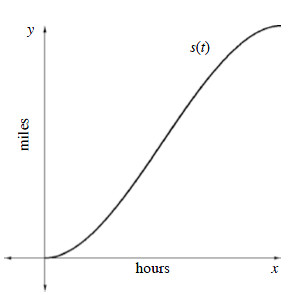While driving to work, Camille decided to keep track of the time and the distance she traveled. Taking the data that she gathered, she found the function s(t) represented her distance as a function of time:
s(t) = −100t3 + 150t2 Homework Help ✎

1. If Camille's trip took 1 hour, how far did she drive?

2. What was her average velocity?

3. Use your graphing calculator to find her maximum velocity. How far into the trip did she reach this speed?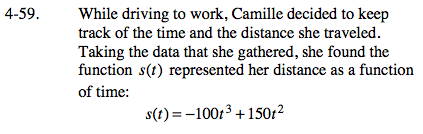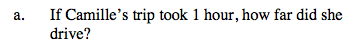You are given the distance function, s(t).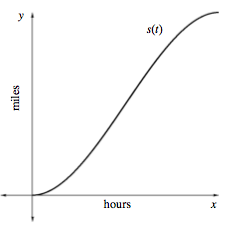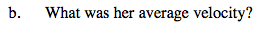In order to let Max Velocity = v(t), you will need the equation for v(t). How can you obtain a velocity equation from a position equation?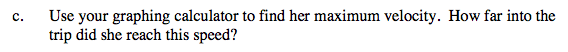If she drove 50 miles in a total of 1 hour, how fast did she travel on average?

Use the eTool below to examine the graph.
Click the link at right for the full version of the eTool: Calc 4-59 HW eTool